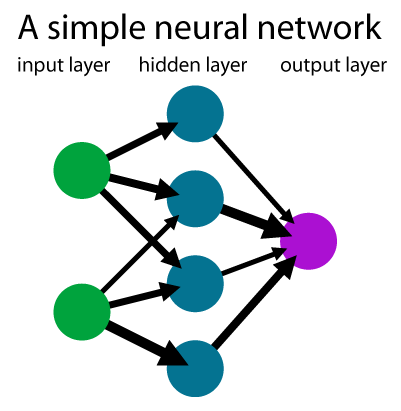# Understanding neural networks: A programmer's perspective

I’ve seen quite a few explanations of neural networks. In the beginning, I found it difficult to make sense of what it was, mechanically. I would often feel like I understood the explanations at the time I was reading them, but it never stuck in my head.

The explanations I’ve seen were either very mathematical in nature, or in an attempt to not sound “mathematical” would present the reader with a variation of this diagram:I know that’s a useful visual for many people, but I’ve always failed to find that diagram useful for myself, and it was always confusing to me. In fact it actually harmed my ability to understand neural networks. Every time I looked at it, I had to think for a while about which things in the diagram represent the activations, and which things represent the weights.

Later on, I discovered that the reason I found that particular diagram difficult was that I was looking at neural networks from the perspective of a computer programmer.

For me, this is a much better diagram:The boxes in the diagram are simply functions. Specifically, these functions:

``````# linear function
def linear(x, W, b):
return np.dot(W, x) + b

# non-linear functions or "activation" functions
def relu(x):
return np.maximum(0, x)

def sigmoid(x):
return 1 / (1 + np.exp(-x))

# ...and others like tanh(x), softmax(x)
``````

That’s all a neural network is. A bunch of layers, where each layer is a linear function `linear(x, W, b)` (`W` and `b` are the learnable parameters at each layer) followed by some non-linear activation function, e.g `sigmoid(x)`. That’s it.

I think this is a much clearer way to think about neural networks, compared to the diagram above, which aims to expand vectors into elements represented as nodes and weights represented as edges.

This new interpretation seems at first glance that it requires knowledge of matrix multiplication and NumPy.

Not really, it doesn’t.

I don’t really care about the algorithm of `linear(x, W, b)` or some non-linear function `nonlinear(x)`. For example, we could have implemented the same linear function using plain arrays, with two nested loops.

The only pertinent thing here is the function signature, that is, what inputs go in, and what I can expect to come out.

Of course, the linear function and non-linear functions have certain mathematical properties that are important to understand.

But these mathematical insights aren’t adequately illuminated by the first diagram either. For example, can you find in the first diagram where the non-linearities are? Neither can I.

By introducting this as a series of functions, it’s far easier to have a top-level understanding of what a neural network actually is.

Once we understand the “bigger picture”, we can then zoom into these functions to examine how each one behaves. So, this is a top-down approach.

I hope this helps people similar to me unblock barriers to understanding.